# Profit and Loss Problems and Solutions for all Competitive Exams

## Questions and Answers on Profit and Loss Chapter | Exercise – 4

In Profit and Loss Exercise – I Covered the terminology with exercise sums on Cost Price (CP), Selling price (SP), Profit percent/Gain percent & Loss percent.

In Profit and Loss Exercise – II Covered the terminology with exercise sums on Mark Up, Marked price/ List Price, Discount, Discount Percentage & Successive discounts.

In Profit and Loss Exercise – III Covered the terminology with exercise sums on Direct Cost, Indirect Cost, Break Even Point, Faulty Measure  or Faulty Balances.. etc

### Now In this exercise given Questions and Answers on Profit and Loss chapter summery of all concepts.

Example – 1 : Naveen Purchased a cycle at cost of ₹2000 and it sold Ram  at 20% profit. Now Ram spent ₹100 for its repair and sold to Jhon at 10% loss. Find the Cost price of Jhon?

Answer: The Cost Price of Naveen = 2000

Sell price of Naveen = 20% profit on 2000

= 2000 x 120 / 100 = 2400

Ram cost price = 2400 + 100 = 2500 (because Ram spent ₹ 100 for its repair )

Sell price of Ram  = 10% loss on 2500

= 2500 x 90 / 100 = 2250

The Cost price of Jhon = ₹ 2250

Example –2 : Jhon sells a article to Tom at a gain of . Tom sells it to Jhosi at a loss of 2 and Jhosi again sells it to Phani at a profit of If  Phani pays to Tom, what is the cost price of the article for Jhon?

Answer : Here take X= +20 , Y = -25, & Z = 20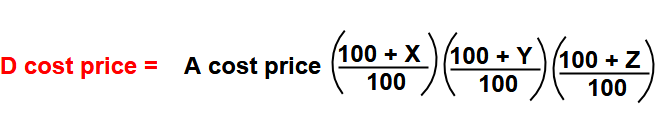Phani cost price ( 1728 ) = Jhon cost price x ( 120 / 100) x (75 / 100)x ( 120 / 100)

Jhon cost price = 1728 x  ( 100 / 120) x (100/75) x (100 / 120) = 1600

Jhon cost price  =

Example – 3 : The Cost price of the two articles were same and  they sold one at 25% profit and another sold at 20% loss. Now find the overall profit/ loss in this transaction?

Answer : One simple formula  while at same cost price

Net profit / loss percent  = ( x + y ) / 2

Here to be take ” +ve” sign for profit and “-ve ” sign for Loss  & same rule also applicable for final answer.

So x = 25 & y = -20

= ( 25 – 20) / 2 =  + 2.5%

Profit = 2.5%

Example – 4 : A person sells two articles at the same price, one at a profit of and another at a Loss of

Answer : For this type of sums we used the below formula  ( while at same selling price)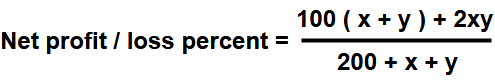Here to be take ” +ve” sign for profit and “-ve ” sign for Loss  & same rule also applicable for final answer.

So  x = 25% and y = – 30%

=[  ( 100 x -5 ) – 1500  ] / 195

= -2000 / 195 = – 400 / 39  (  – ve sign means it is Loss )

Example -5 : A shopkeeper sells 20% articles at 20% profit and he sells 30% of remaining articles at 25% loss. Now remaining two articles sold at 50% profit.

Answer : Here Take articles = 100 and Cost Price = 100

Transaction – 1 – Sells 20% articles at 20% profit

= 20 x 120 / 100 = 24

Transaction – 2 – Sells 30% of remaining articles at 25% loss.

So 30% of remaining articles means 30% of 80

= 80 x 30 / 100 = 24 articles at 25 % loss

=24 x (75 / 100)

= 18

Transaction – 3 – Remaining Sold at 50% profit.

Remaining articles 56 (=100 – 20 – 24) sold at 50% profit

= 56 x 150 / 100 = 84.

The total sold price of 100 articles = 24 + 18 + 84 = 126

So In this overall transaction the shopkeeper got 26% profit.

Example – 6 : A shopkeeper offer successive discounts 60% , 20% & 20% on product. Now find the overall discount on that product

Answer : Here take d1 = 60% , d2 = 20% & d3 = 20% and use this in following formulas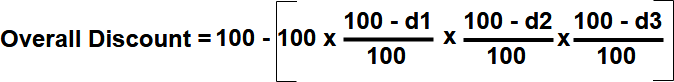= 100 – [ 100 x (40 /100 ) x ( 80/100) x ( 80 /100) ]

= 100 – 25.6

= 74.4 %

Overall Discount = 74.4 %

Example – 7 :  A company sells two goods for 24000 each. One it gained 25% and on the other it lost 25%. What is the overall gain or loss percent?

Hint: If SP of two articles is same such that there is x% gain on one and x% loss on the other then there will be always a loss of  ( x / 10 )2.

So here x = 25

= (25 / 10 )2

= 625 / 100 = 6.25%

Loss percent = 6.25%.

Example – 8 : A Shopkeeper marks his goods 20% above the cost price , but allows a discount of 10% on the marked price. What is the gain or loss% in the deal for the shopkeeper?

Hint : If there is two successive changes  x% and y% then overall change in percent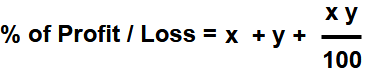Here to be take  “-ve” sign for loss/ discounts and +ve sign for profit / mark up and finally the value comes ” +ve ” then it  gives profit and the values comes ” -ve ” value then it incurred Loss.

sao x = + 20 , y = – 10

% of profit / Loss = 20 – 10 – ( 200 / 100) = + 8%

Profit percent = 8%.

Example – 9 : A Shopkeeper even after allowing a discount of 20% , he still gets a profit of 30% on the article. Then find mark up percent of that article ?

Answer: Here also using the above formulax = ? , y = -20, %profit = 30

30 = x – 20 – (20x / 100)

50 = 0.8 x

x = 500/8 = 62.5

Marked percent of the article = 62.5%

Example – 10 :  A fruits vender  cost price of apples is equal to selling price of apples, what is the profit/loss percentage?

Answer : Here using the following formula

If cost price of “ “objects is same as selling price of “ objects, profit / loss percentage

[ ( x – y ) 100 ] / y

Now x = 20 and y = 15

Profit / loss percent = [ ( 20 – 16) 100 ] / 16 = 400/16 = + 25% ( “+ve sig means profit and -ve sign means loss)

Profit percent = 25%

Example – 11 :  A trader sells his product at loss and he uses less weight. Find his overall profit/loss percentage.

Answer : Take   m = 100  ,  (m – t) = 25 , P = -20 , t = 75 , (100% – 25%)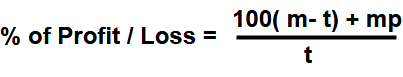Profit /loss % =  ( 100 x 25 – 20 x 100 ) / 75 = 500 / 75  = 20 /3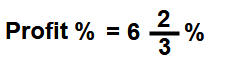Quantitative Aptitude Main Page

Shortcut of Math Tricks

## 2 thoughts on “Profit and Loss Problems and Solutions for all Competitive Exams”#### vinay

(November 4, 2018 - 3:05 pm)

Can you please chick example 6 (near 200+x+y), According to given values x=25,y=-30 so (200+25-30 = 195) its not 255#### sivaalluri

(November 6, 2018 - 3:49 pm)

Thank you for your valuable correction